### Home > MC2 > Chapter 1 > Lesson 1.1.3 > Problem1-19

1-19.

Find the perimeter of each polygon below. Note that the markings on part (e) show that all sides are the same length. Show your work.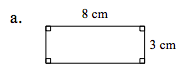This shape is a rectangle. Find its perimeter. Remember that the perimeter is the total length of the border about the shape.

Find the unknown lengths and label them on your diagram. Now try to find the perimeter without looking at the answer.

22 cm

Be sure to show why the perimeter is 22 cm.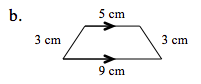The perimeter is the total length of the border about the shape.

The perimeter is 3cm + 5cm + 3cm + 9cm.

Calculate this sum.

20 cm.

Can you show how you arrived at this answer?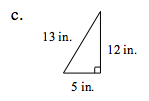See (a) and (b).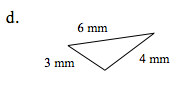See (a) and (b).

13 mm.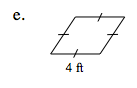The markings mean that all the sides are 4 ft long.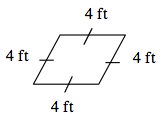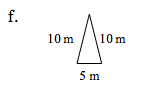See (a) and (b).